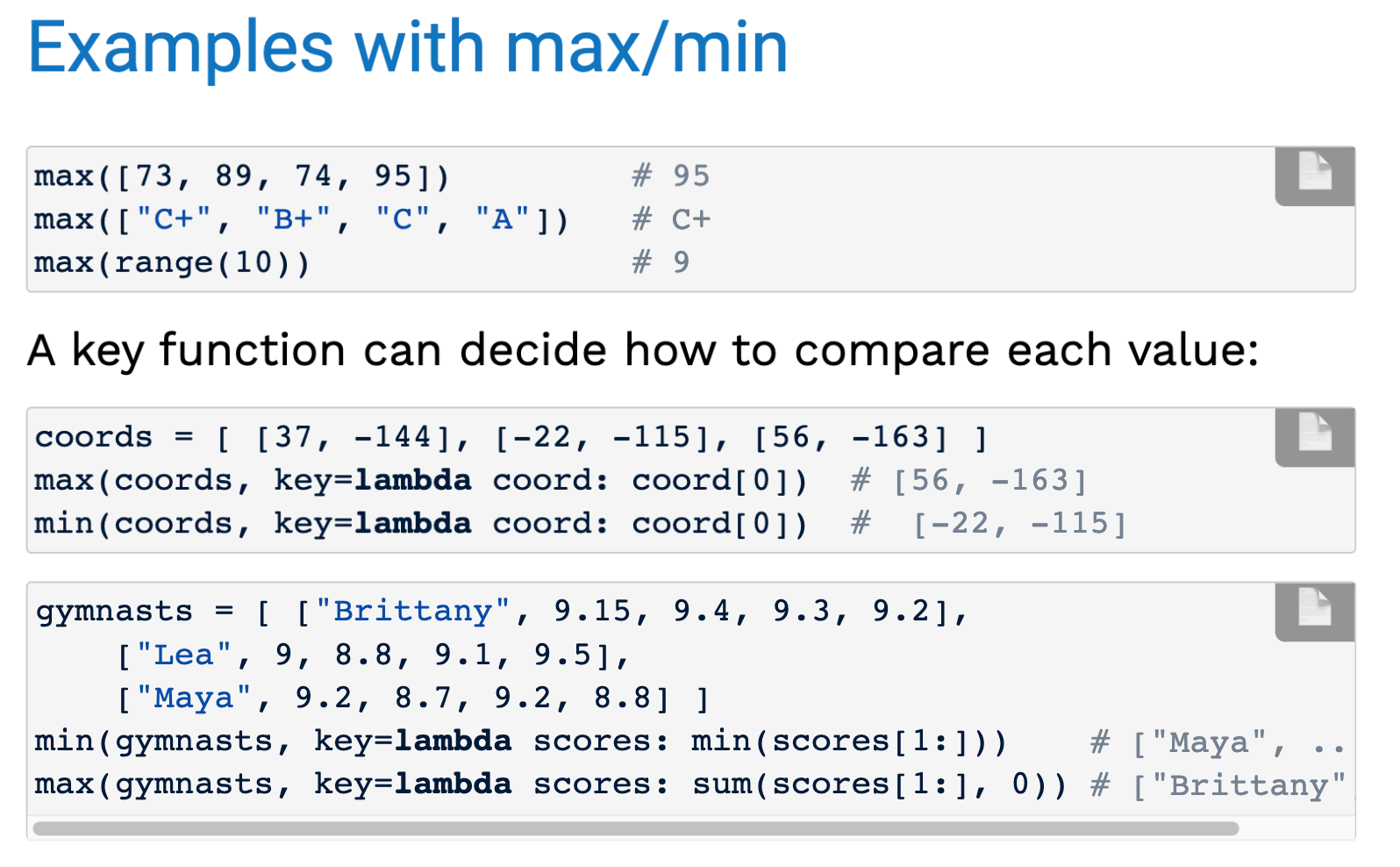﻿ 柴柴のSequences Data abstraction

### 柴柴のSequences Data abstraction

2021-10-28 7:25:03

# 1. Sequences

Copy a list

listC = list(listA) / listA[:]

``````def sum_nums(nums):
"""Returns the sum of the numbers in NUMS.
>>> sum_nums([6, 24, 1984])
2014
>>> sum_nums([-32, 0, 32])
0
"""
if (nums == []):
return 0
else:
return nums + sum_nums( nums[1:] )``````

When recursively processing lists, the base case is often the empty list and the recursive case is often all-but-the-first items.

If a recursive function needs to keep track of more state than the arguments of the original function, you may need a helper function.# 2. Data abstraction

data abstraction lets us manipulate compound values as units, without needing to worry about the way the values are stored. 拆分compound

### A pair abstraction

If we needed to frequently manipulate "pairs" of values in our program, we could use a `pair` data abstraction.

 `pair(a, b)` constructs a new pair from the two arguments. `first(pair)` returns the first value in the given pair. `second(pair)` returns the second value in the given pair.

### Rational numbers

 Constructor `rational(n,d)` constructs a new rational number. Selectors `numer(rat)` returns the numerator of the given rational number. `denom(rat)` returns the denominator of the given rational number.

### Reducing to lowest terms

`from math import gcd def rational(n, d): `

`"""Construct a rational that represents n/d in lowest terms.""" `

`        g = gcd(n, d) `

`                return [n//g, d//g]`